Libri di Michele Zenga - libri Statistica & Applicazioni (2)

# Michele Zenga

### Titoli dell'autore

First applications of a new three-parameter distribution for non-negative variables
Free
digital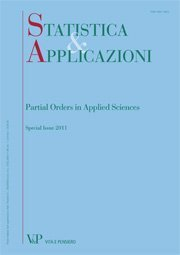Year: 2012
SUMMARY Zenga (2010a) recently proposed a new three-parameter family of density functions for non-negative variables. Its properties resemble those of economic size distributions: it has positive asymmetry, Paretian right tail and it may be zeromodal, unimodal or even bimodal. In this paper we explore some methods for fitting the new density to empirical income distributions. We will see that D’Addario’s invariants method clearly outperforms Pearson’s moments method, which does not seem to work well with heavy tailed distributions. Further, we propose some new methods based on the minimization of a measure for the goodness of fit, imposing restrictions on the parameter space to preserve some features of the empirical distribution in the fitted model. We will see that these methods provide very satisfactory results with income distributions from Italy, Swiss, US and UK. Keywords: Income Distribution, Zenga’s Distribution, Goodness of Fit, Moments Method, Invariants Method.
Point and interval estimation for some financial performance measures
Free
digitalYear: 2011
We study the estimators of three financial performance measures: the Sharpe Ratio, the Mean Difference Ratio and the Mean Absolute Deviation Ratio. The analysis is performed under two sets of assumptions. First, the case of i.i.d. Normal returns is considered. After that, relaxing the normality assumption, the case of i.i.d. returns is investigated. In both situations, we study the bias of the estimators and we propose their bias-corrected version. The exact and asymptotic distribution of the three estimators is derived under the assumption of i.i.d. Normal returns. Concerning the case of i.i.d. returns, the asymptotic distribution of the estimators is provided. The latter distributions are used to define exact or asymptotic confidence intervals for the three indices. Finally, we perform a simulation study in order to assess the efficiency of the bias corrected estimators, the coverage accuracy and the length of the asymptotic confidence intervals. Keywords: Financial Performance Measure, Sharpe Ratio, Mean Difference Ratio, Mean Absolute Deviation Ratio, Concentration Measures, Statistical Analysis of Financial Data.
More on M.M. Zenga’s new three-parameter distribution for nonnegative variables
Free
digital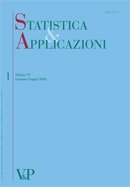Year: 2011
SUMMARY Recently Zenga (2010) has proposed a new three-parameter density function f (x : µ; α; θ), (µ > 0; α > 0; θ > 0), for non-negative variables. The parameter µ is equal to the expectation of the distribution. The new density has positive asymmetry and Paretian right tail. For θ > 1, Zenga (2010) has obtained the expressions of: the distribution function, the moments, the truncated moments, the mean deviation and Zenga’s (2007a) point inequality A(x) at x = µ. In the present paper, as to the general case θ > 0, the expressions of: the distribution function, the ordinary and truncated moments, the mean deviations and Zenga’s point inequality A (µ) are obtained. These expressions are more complex than those previously obtained for θ > 1 by Zenga (2010). The paper is enriched with many graphs of: the density functions (0.5 ≤ θ ≤ 1.5), the Lorenz L(p) and Zenga’s I (p) curves as well as the hazard and survival functions. Keywords: Non-Negative Variables, Positive Asymmetry, Paretian Right Tail, Beta Function, Lorenz Curve, Zenga’s Inequality Curve, Hazard Function, Survival Function.
Mixture of Polisicchio’s truncated Pareto distributions with beta weights
Free
digitalYear: 2010
A new three-parameter density function f ðx : ; ; Þ for non-negative variables, obtained as a mixture of ‘‘Polisicchio’s truncated Pareto distributions’’, is proposed. The expectation of f ðx : ; ; Þ is equal to the parameter  > 0. The new density has positive asymmetry and Paretian right tail. The variance is equal to 2 3 ð þ 1Þ ½2 þ ð  1Þ   . The moments, the upper and lower truncated moments (with truncation at x ¼ ), are compact expressions of beta functions. Keywords: Income Distribution, Positive Asymmetry, Paretian Tail, Mixture Density, Beta Weights
Measuring loan recovery rate: methodology and empirical evidence
Free
digitalYear: 2008
This paper aims at proposing a new methodology to compute recovery rate on non-performing bank loans, in order to confine this variable within the interval [0,1]. Such a methodology is then applied to data on loans gathered by the Bank of Italy and some interesting characteristics of the loan recovery process in the Italian banking market are highlighted. The combined effects of some variables on the recovery rates are also analysed. In particular, the presence of either collateral or personal guarantee, the borrower’s residence area are considered, thereby emphasizing the relationship between the recovery rate and the total exposure.
Asymmetry for ordinal variables
Free
digitalYear: 2007
This paper proposes, for ordinal variables, an index of asymmetry based on the cumulative and retrocumulative frequencies. The paper shows that this new index has a connection with the bipolar mean, recently introduced by Maffenini and Zenga (2006). An application to a real case is presented to show how the asymmetry index works.
Inequality curve and inequality index based on the ratios between lower and upper arithmetic means
Free
digital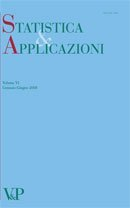Year: 2007
A new inequaliy curve I(p) based on the ratio between the lower mean M(p) and the upper mean þ M+(p) is proposed. By averaging I(p) the new inequality index I is obtained. The index I satisfies the usual properties required to an inequality measure. Being U(p)=1-I(p) a ratio between two arithmetic means, the meaning of I(p) is very straightforward. The index I is related to the Gini index R and the Bonferroni index B by the relation: R
Editorial digital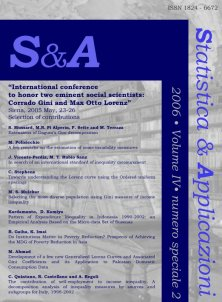Year: 2006
This second special issue of Statistica & Applicazioni provides a selection of papers, which were presented and discussed at the International Conference to Honor Two Eminent Social Scientists.
Convergence of the Sample Mean Difference to the normal distribution: simulation results digital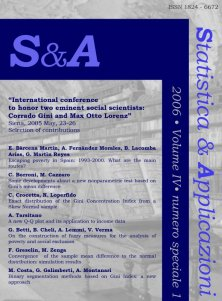Year: 2006
The present work aims to obtain the value of minimum sample size required by a good approximation by the normal curve for the sample mean difference. Particular care is given to what happens in the tails of the curves, with the aim of deriving confidence intervals for Gini’s mean difference. This goal is obtained by empirical methods and the presented results have an explorative nature. Simulation data have been obtained sampling from different distributions, considering symmetry versus asymmetry and the existence of the moments as main aspects in the underlying distribution. These remarks lead to the choice of the normal, the rectangular, the exponential and the Pareto distributions. All the obtained results indicate that the shape of the distribution from which the samples are generated is critically related to the minimum sample sizes required for a good approximation of the tails of the sample mean difference to the normal curve. Keywords: Gini Mean Difference, asymptotic distribution, convergence, U-statistic.
Editorial digitalYear: 2006
This special issue of Statistica & Applicazioni provides a selection of papers, which were presented and discussed at the International Conference to Honor Two Eminent Social Scientists.
Bipolar mean for ordinal variables digital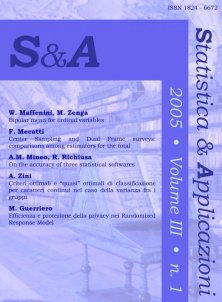Year: 2005
This paper proposes, for ordinal variables, a new type of mean called bipolar mean, that is a frequency distribution with the total size n concentrated on one category or on two consecutive categories.